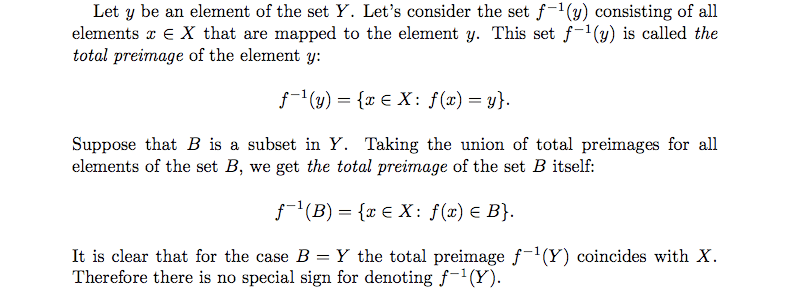# Casey's thread of annoying Linear Algebra questions

In summary, this person is trying to learn linear algebra on their own and is using a book by Sergei Treil. They have a question about what they need to know in order to start and people have given them helpful advice.

So, I am attempting to learn linear algebra on my own and I am using Sharipov's "Linear Algebra and Multidimensional Geometry" since mathwonk is always ranting and ravong about it.

So far, I hate it. Clearly I am not a mathematician at heart.

I thought I could periodically post questions in this thread (instead of starting a new one every time). My questions might seem dumb to some of you, but take it easy on me:shy:.

So since the edit button only works for 30 minutes now (lame), when I ask a new question, I will head it like this: NEW QUESTION: that way it will be easy to spot my latest entry.

Hopefully this works out, but more like is that people will just get annoyed with me. So here we goNEW QUESTION What is this all about?I mean if the set $f^{-1}(y)$ is just X, why do we need a name for this? Can someone tell me what the total preimage is in laymen's terms?

Thanks!http://img253.imageshack.us/img253/2554/preimagery4.png [Broken]

Last edited by a moderator:
learning math is not meant to be suffering. use whatever book you enjoy. try the book by sergei treil on his webpage at the brown math dept, if sharipov is too dry for you.

enjoy!

and the total preimage (under f) of a point y is the set of all solutions x of y = f(x).

so finding a total preimage is finding all solutions of a problem.

One place where the idea of a preimage is useful is in the definition of a continuous function: A function $f:X\rightarrow Y$ is said to be continuous if $f^{-1}(U)$ is an open set for every open $U\subset Y$.

If $X=Y=\mathbb R$ (or more generally, when X and Y are metric spaces), then the definition above is equivalent to the one you're familiar with.

Also, the preimage of y is not X. For example, if $f:\mathbb R\rightarrow\mathbb R$ is defined by $f(x)=x^2$, then $f^{-1}(1)=\{-1,1\}$.

mathwonk said:
learning math is not meant to be suffering. use whatever book you enjoy. try the book by sergei treil on his webpage at the brown math dept, if sharipov is too dry for you.

enjoy!

and the total preimage (under f) of a point y is the set of all solutions x of y = f(x).

so finding a total preimage is finding all solutions of a problem.

Thanks! I'll check that one out and see how it is.hello all I also have an annoying question, I also d like to attempt to learn some linear algebra on my own, I was wondering what do I need to previously know in order to start learning it? Do I need any calculus or it would be ok with just to know high school algebra?

You don't need calculus. You need to understand what functions are and have some idea what sets are to understand the definition of a vector space, and you need know how to add and multiply real numbers (e.g. rules like (a+b)(c+d)=ac+ad+bc+bd). But that's it.

Fredrik said:
You don't need calculus. You need to understand what functions are and have some idea what sets are to understand the definition of a vector space, and you need know how to add and multiply real numbers (e.g. rules like (a+b)(c+d)=ac+ad+bc+bd). But that's it.

thanks a lot